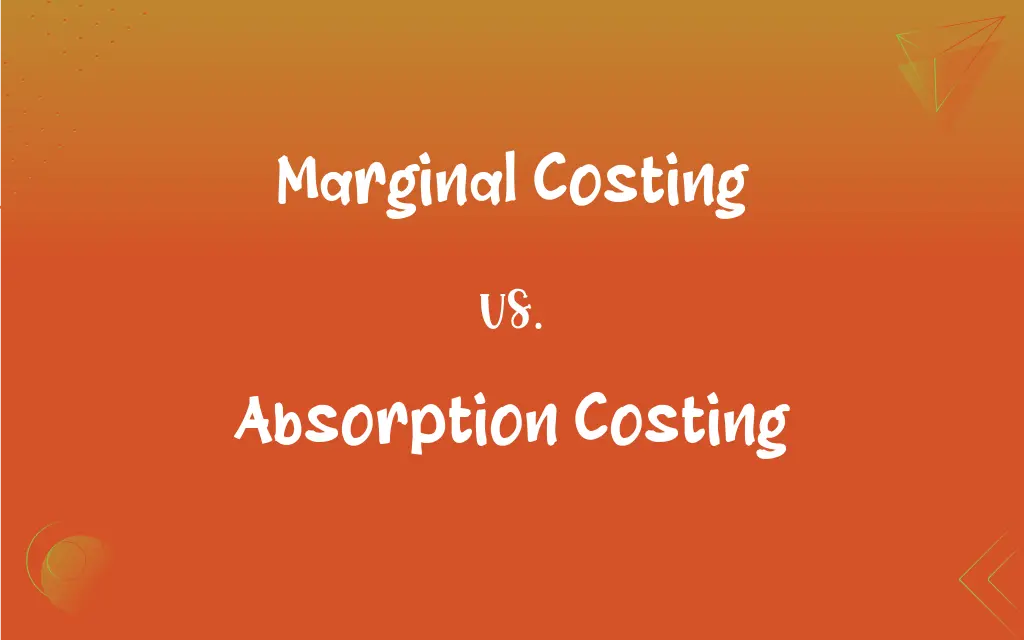# Marginal Costing vs. Absorption Costing

The main difference between Marginal Costing and Absorption Costing is that Marginal costing doesn't take or bring fixed costs into account under product costing or inventory valuation. Absorption costing, on the other hand, takes both fixed costs and variable costs into account.## Key Differences

In Marginal Costing, Product relevant costs will comprise only variable cost while in the case of Absorption costing, fixed cost is also comprised of product-related cost apart from variable cost.
In marginal costing profit can be determined through the help of Profit Volume Ratio [(Contribution / Sales) * 100]. On the other hand, Net Profit displays the profit whether Absorption Costing.
Marginal Costing serves overheads into two broad categories, i.e., Fixed Overheads and Variable Overheads. Look at the other condition Absorption costing, which categorizes overheads in the following three categories Production, Administration and Selling & Distribution.
In Marginal Costing conflicts in the opening and closing stock will not affect the per unit cost. Unlike Absorption Costing, where the conflicts between the stock at the beginning and the end will show its outcome by increasing/decreasing per unit cost.
The costing procedure in which varying cost apportioned exclusively, to the commodities is known as Marginal Costing. Absorption Costing is a costing procedure in which all the costs are absorbed and apportioned to products.
In marginal costing, the cost data is provided to outline the total cost of every outcome. Whereas, in absorption costing, the cost data is presented traditionally, the net profit of each product ascertained after deducting fixed cost along with the variable cost.

## Comparison Chart

### .

A decision-making skill for determining the total cost of production is known as Marginal Costing.
Allocation of total costs to the cost center to determine the total cost of production is known as Absorption Costing.

Fixed and Variable
Production, Administration and Selling & Distribution

### Cost Per Unit

Variances in the opening and closing stock do not influence the cost per unit of output.
Variances in the opening and closing stock affect the cost per unit.

### Cost Data

Presented to outline the total contribution of each product.
Presented conventionally.

### Profitability

Profitability measure by Profit Volume Ratio.
Due to the addition of fixed cost, profitability gets affected.

### Highlights

Contribution per unit
Net Profit per unit

### Marginal Costing vs. Absorption Costing

Marginal costing doesn’t take fixed costs into record or account under product costing or inventory evaluation. Absorption costing, on the other hand, gets both fixed costs and variable costs into the record. Marginal costing classified as fixed costs and variable costs whereas Absorption costing classified as production, dispersion, and selling & administration. Marginal costing expressed as contribution per unit and Absorption costing expressed as net profit per unit. Marginal costing is a method of cost, and it isn’t a conventional way of looking at costing method. Absorption costing, on the other hand, is used for financial and tax reporting and it is the most conventional method of costing.

The profitability of each sale will appear to be higher under marginal costing, while profitability will appear to be lower under absorption costing. The measurement of profits under marginal costing uses the contribution margin (which excludes applied overhead), while the gross margin (which includes applied overhead) is used under absorption costing. Marginal costing is not allowed for financial reporting purposes, so its use restricted to internal management reports whereas the applicable accounting frameworks require absorption costing for financial reporting purposes so that that factory overhead included in the inventory asset.

### What is Marginal Costing?

Marginal Costing, also termed as Variable Costing, is a costing method by which decisions are taken regarding the determination of total cost or the determination of the fixed and variable cost to find out the best method and product for production, etc. It determines the Marginal Cost of production and shows its effect on profit for the change in the output units. Therefore, the fixed cost of production posted to the Profit & Loss Account. Moreover, fixed cost is also not given pertinence while determining the selling price of the product or at the time of evaluation of closing stock.

#### Features

• Cost Classification: The marginal costing method makes a sharp distinction between variable costs and fixed costs. It is the varying cost based on which a firm designs production and sales policies following the marginal costing technique.
• Stock/Inventory Valuation: Under marginal costing, inventory for profit measurement valued at marginal cost. It is in strong contrast to the total unit cost under absorption costing method.
• Marginal Contribution: Marginal costing procedure makes use of a marginal contribution for making various The marginal contribute is the difference between sales and marginal cost.

### What is Absorption Costing?

Absorption Costing is a procedure for inventory valuation whereby all the manufacturing expenses are assigned to the cost centers to identify the total cost of production. These manufacturing expenses comprise all fixed as well as variable costs. In an absorption costing system, together the fixed and variable costs regarded as product related cost. In this method, the objective of the allocation of the total cost to cost center is to retrieve it from the selling price of the product. Based on function, the expenses divided into Production, Management, and Selling & Distribution.

#### Types

• Activity Based Costing
• Job Costing
• Process Costing

#### Components

• Direct production costs or prime costs: (i) Direct materials, (ii) Direct labor, (iii) Direct expenses.
• Indirect production costs/ overheads: (i) Indirect materials, (ii) Indirect labor, (iii) Factory rent, (iv) Depreciation of plant, (v) Factory cleaning.